May 22, 2020
5 Views
0 0

# Using elementary mathematics to close holes of even a very complex shape

No one knows how to build on the plane the smallest figure that can cover all other figures of a certain width. But the geometry of high school allows you to get closer to solving this problem.

Suppose you have an amazing needle and thread. Your friend, knowing this, wrote you a letter asking for help: “Hey, I have jeans that are leaky. Can you patch them for me? ”

"Of course! It’s easy, you answer. “And what size holes?”

“They are all different and irregular in shape, but not wider than an inch.” I'll drop in a little later, so get ready! ”

You take out your sewing kit and take out a few round pieces of fabric, each 1 inch in diameter. “That should do,” you think. But is it suitable? Is a round patch with a diameter of 1 inch really able to cover any hole with a width of no more than 1 inch in any direction?

You take from your set a piece of fabric in the form of an equilateral triangle with one inch sides. Between the two points in this triangle, you note, the distance is not more than 1 inch, so some of the holes in your friend's jeans may well have the same shape. But, having superimposed on a triangle one of the rounds you have prepared, you notice that the round patch covers only two vertices of the triangle, and the third remains open.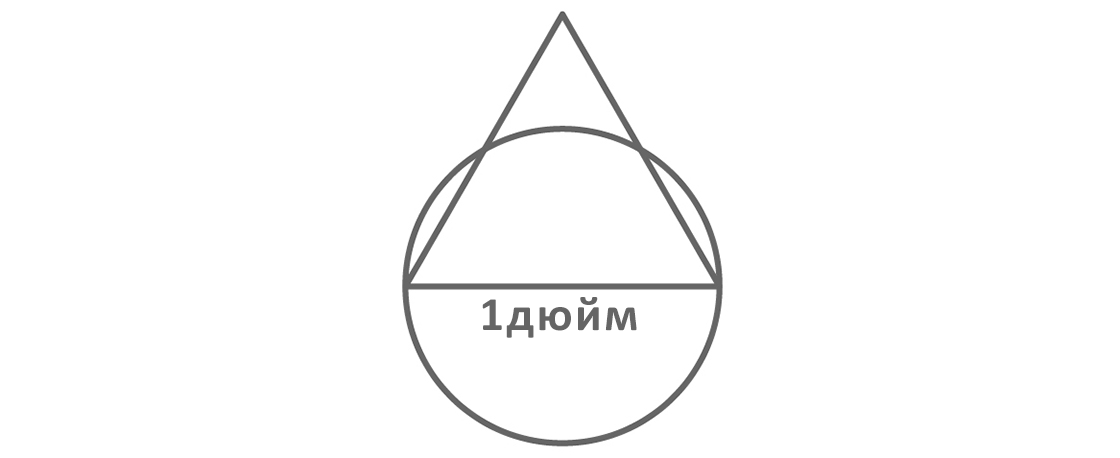The fact is that, according to elementary geometry, the height of this triangle$displaystyle{frac{sqrt{3}}{2}}$ inches, that is, more than the radius of a given circle ($displaystyle{frac{1}{2}}$ inches)). A circle cannot close a triangle, and a triangle cannot close a circle. Since the hole can have any shape, it turns out that none of the pieces of fabric you have prepared can guarantee that any hole in your friend's jeans will be closed.

You can simply take such a big round that guarantees you success, but you don’t want to waste precious material. Therefore, the question arises: what should be the smallest piece of fabric that can close a hole with a width of no more than 1 inch? By looking for an answer online, you will find out that mathematicians are also pondering this question: they are more minimum patch. This ideal form has not yet been found, but thanks to recent studies, we have been able to advance significantly.

For the first time, the “universal coverage problem” was posed in 94 by Henri Lebesgue in his letter to fellow mathematician Julius Pál. The problem can be formulated in different ways, but it is based on the concept of "area of ​​unit diameter." Here we have in mind the set of points on the plane in which there are no two points separated by more than 1 unit. In our problem of fixing pants, this is a hole no wider than 1 inch.

If one set of points can fit inside another, they say that the second set “covers The first is like a patch covering a hole. A “universal coating” is a region that is capable of covering all forms of unit diameter, and in order to solve the problem of a universal Lebesgue coating, it is required to obtain the smallest convex region. (“Convex”, if we explain it loosely, means that the desired coverage does not have any recesses, and the “smallest” - “with a minimum area”).

You may be surprised what has passed 61 years, and this geometric problem, which seems to be elementary, to solve failed to. However, in fact, the Lebesgue problem is by no means simple, and partly because it is difficult to accurately depict the desired shape of a unit diameter. As we know, the proof of theorems on the properties of an object that cannot be clearly presented can be very difficult.

To cover all sets of unit diameter, we need “ patches "of suitable forms. We know many such forms, but none of them are the smallest. Let’s find out why mathematicians are unable to tailor the “patch” of the desired shape.

Let's start with the area R of unit diameter. We have no idea what R looks like; we just know that, like the holes that need to be closed by this area, its width does not exceed unity. However, since it has a unit diameter, suppose that it includes the points A and B, the distance between which is 1 unit.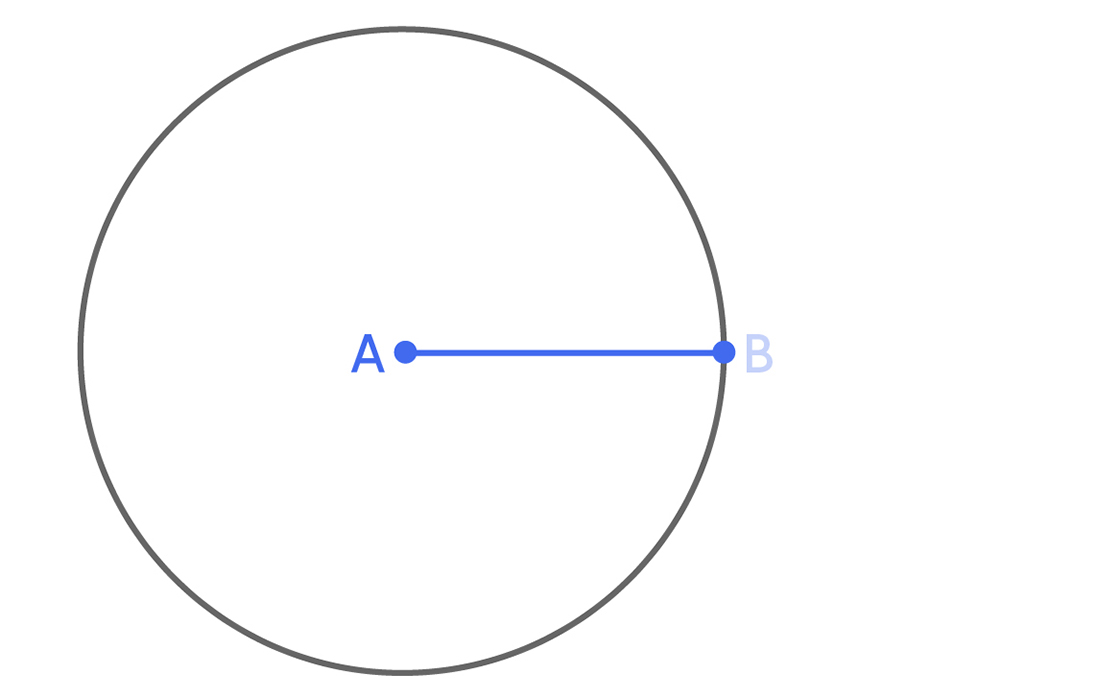Now suppose that in R, the dot also C. Where can be located C Distance from C to A cannot be more than single, therefore, the point C should be within the circle of a unit radius centered in A . You can build this circle with a compass - centered in Aand a circle passing via B.But the distance from from to In , too, there can be no more than a single, so the point C must be within the circle of unit radius centered at B . This circle can also be built using a compass.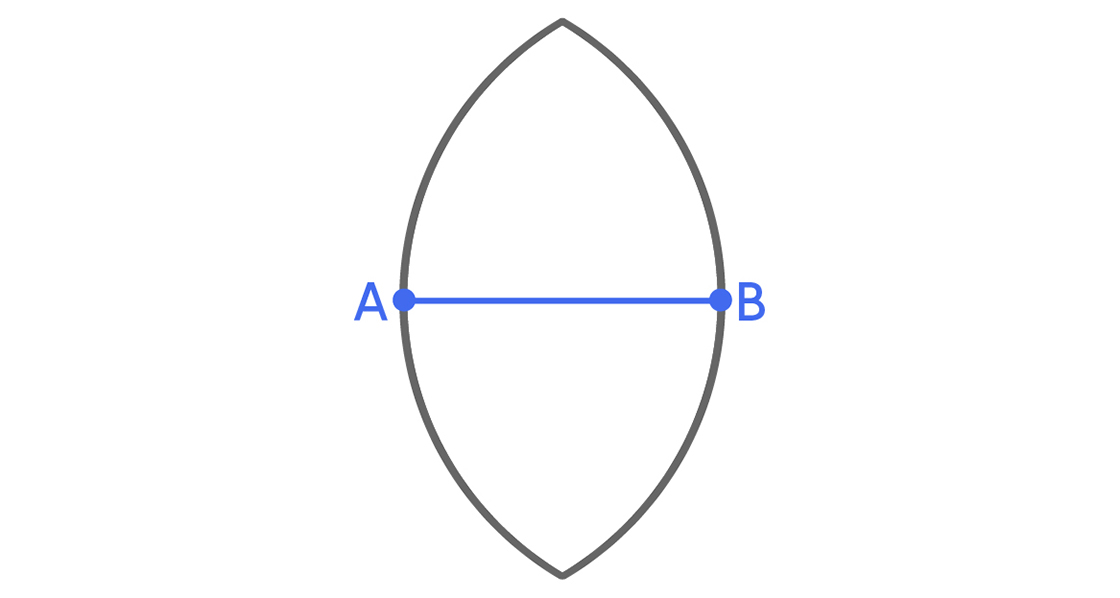So, the dot C must lie at the intersection of these two circles.This reasoning applies not only to the point WITH; it applies to all points in R. Thus, any point in R must belong to the intersection area of ​​the two circles we constructed. In other words, this region can cover all possible sets Rof unit diameter and is a universal coating .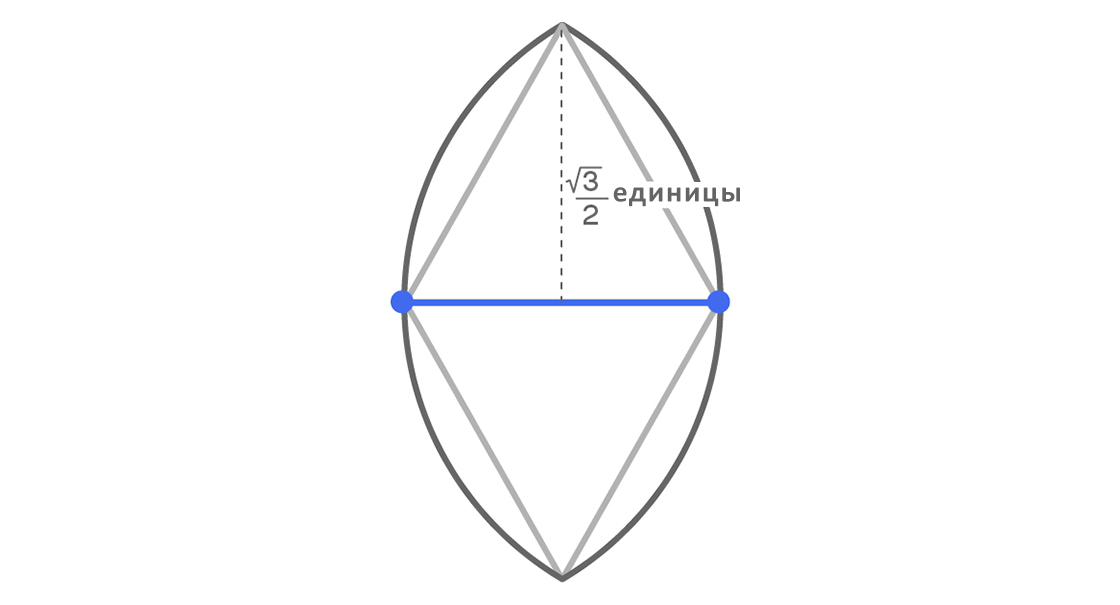But this universal coverage is not the smallest. Let's try to trim it.

Note that the points of the intersection of the circles form two equilateral triangles with A and B , and the distance from the top point (and from the bottom) to the middle of the segment AB is$displaystyle{frac{sqrt{3}}{2}}$ units.As$displaystyle{frac{sqrt{3}}{2} > frac {1} {2}}$, we can draw parallel lines at a distance of$displaystyle{frac{1}{2}}$ units from$displaystyle{overline{AB}}$ on both sides, as shown below.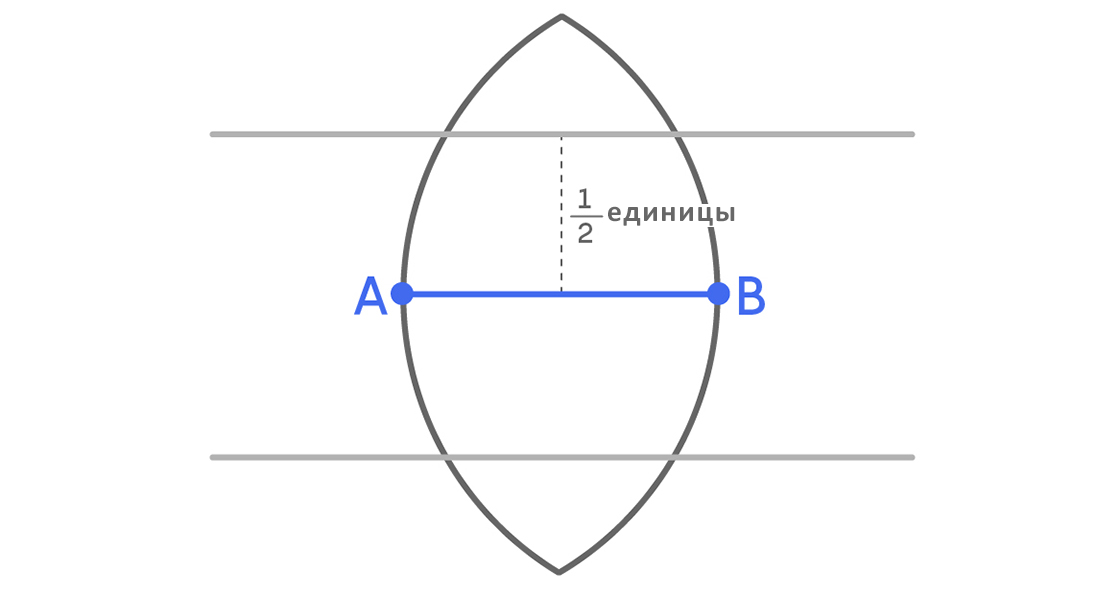Now consider two areas of red color, one of which is above the upper parallel line, and the other is below the bottom.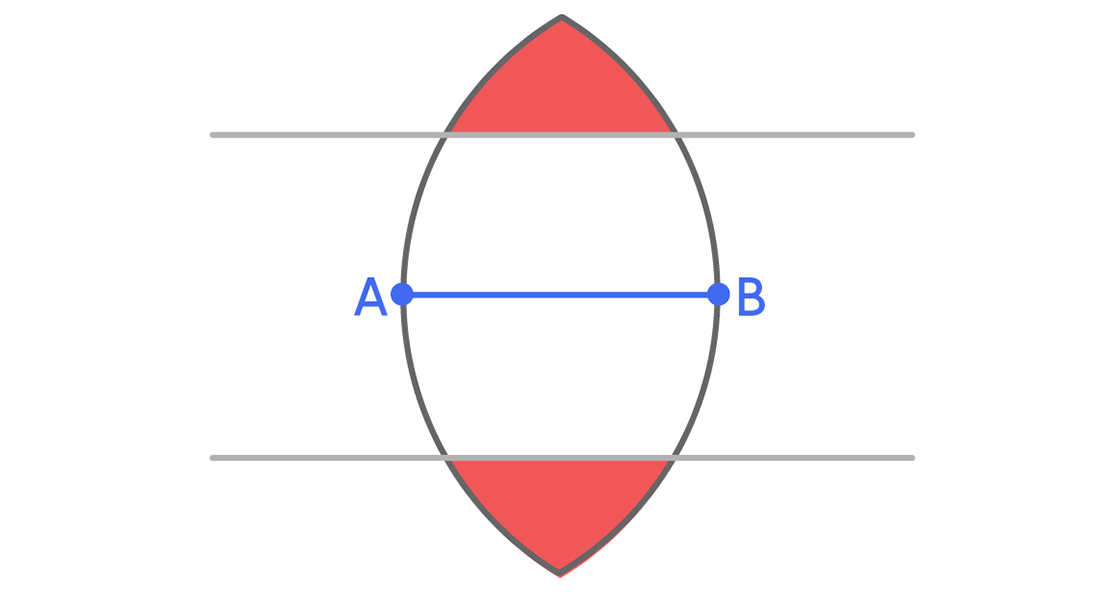Since the distance is m Between two parallel lines is equal to one, a set of unit diameter cannot simultaneously be in both red areas. This means that for the universal coverage, both red areas are not needed. We can simply delete one.$displaystyle{frac{2pi}{3}-frac{sqrt{3}}{2} approx {1,228}}$

The coating we originally obtained - the area of ​​intersection of two circles - has an area$displaystyle{frac{pi}{2}-frac{1}{2} approx {1,071}}$, and the result now is the area. Starting with the simplest universal coverage, we were able to make it smaller by removing the extra part.

That's how mathematicians came to our version of the smallest universal coverage. Using more advanced methods, we can find other simple forms in order to improve them later. For example, it can be shown that a universal covering is a square with a side equal to one. And Pal, solving the Lebesgue problem, used the properties of the so-called "curves of constant width." Using these curves, he showed that even if some part of the set of unit diameter "crawled out" outside the circle of unit diameter that covers it, it can always be shifted or rotated so that it is completely covered by the hexagon into which this circle is inscribed:$displaystyle{frac{sqrt{3}}{2} approx {0,866}}$

The following is shown as the hexagon of Pal closes the unit diameter of three different shapes. The shape in the middle is the Reuleaux triangle, a curve of constant width, closely related to the coating examples we examined above. (In order to construct the Röhlo triangle using our examples, it is necessary to draw an arc of unit radius from A from the upper point of the intersection of two circles to B).This hexagon has an area, which is less than the area of ​​our coatings and the square with a single side. However, in addition, Pal showed that we do not need the entire hexagon. Arguing as described below, he found some extra parts that could be cut off.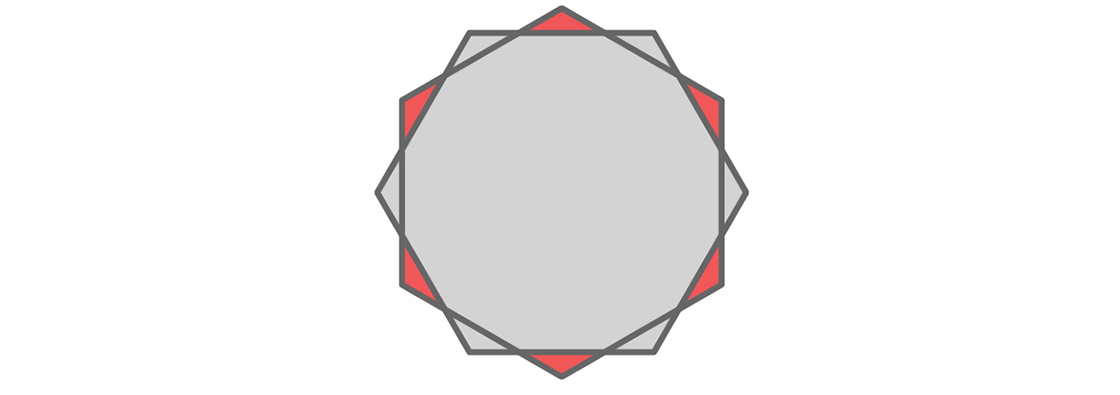and turn one of them to 94 degrees around their common center.Thanks to this, a lot of interesting things appear - for example, the intersection area of ​​two hexagons is a dodecagon - but we are most interested in six small red triangles shown below.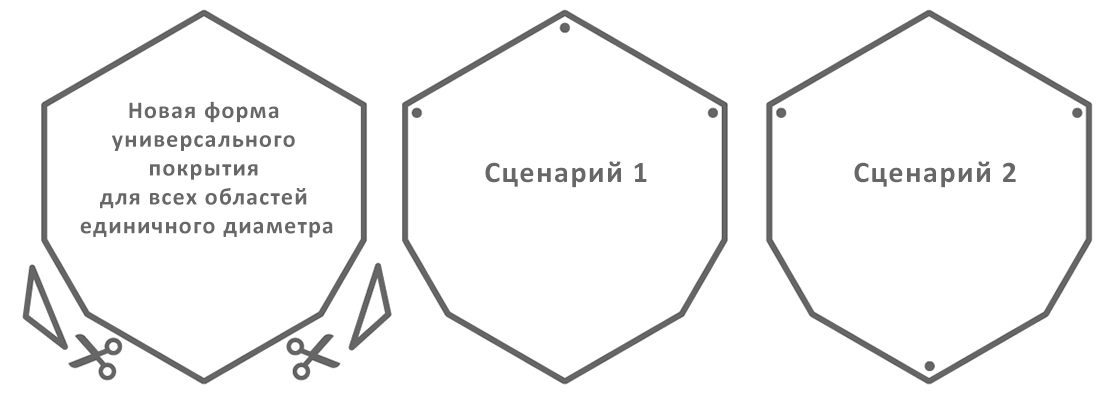Each red triangle is inside the hexagon that we did not rotate, and outside the rotated hexagon. Since the distance between any two opposite sides of both hexagons is one, the distance between points inside two opposite red triangles must be greater than one. As in our discussion above, a universal coating does not need both triangles from each pair of opposing triangles, since a set of unit diameter cannot be simultaneously in both. Therefore, we have the opportunity to remove some triangles, and, apparently, there will be three (one from each pair). But, unfortunately, by removing the three red triangles, we will deprive our coverage of the ability to close any sets of unit diameter. Let's find out why.

So that the hexagon does not undergo any changes during rotation, you can turn it on 94 degrees or along one of the symmetry axes, therefore, in reality, there are only two ways to choose one red triangle from each pair of triangles opposite each other: you can take three consecutive triangles or every second of six triangles. This is shown below. The dots indicate those triangles that can take up a lot of unit diameter.If there are many , which should be covered, occupies three consecutive triangles, as shown on the left, it will not be able to close the cover of the form that is obtained by removing every second of six triangles, as shown on the right. And if this set occupies every second of the six triangles, it cannot be covered by a coating having the shape obtained by removing three consecutive triangles. Removing any set consisting of three triangles leaves the possibility that some set of unit diameter will not be closed. Therefore, we cannot remove the three red triangles.

But we can remove the two. The two problem sets described above can still be closed if we remove two red triangles that are neither adjacent nor opposite. That's exactly what Pal did.$displaystyle{frac{2}{sqrt{3}} approx {0,8453}}$

To get a new form that completely covers any area of ​​unit diameter, he cut two triangles from his hexagon. This new universal coating has a slightly smaller area than the hexagon: 87763.

On this, cutting off the excess pieces did not end. Smaller pieces were successfully removed by the mathematicians Roland Sprague and H. Hansen - in 1120 and 1120, respectively Just a few years ago, amateur mathematician Philip Gibbs suggested cutting off a few new pieces. He was inspired by the publication of mathematician-blogger John Baez. Together with Baez and another mathematician, Gibbs summarized the methods of Sprague and Hansen to further reduce the coverage. As a result, a new world record was set in creating the smallest convex coating for sets of unit diameter. Gibbs himself quickly improved on this achievement, additionally removing unnecessary areas.

It’s great that cutting off the extra pieces from the Pala hexagon continues, but only these pieces have become tiny. German Sprag reduced coverage by approximately 0, 94 square unit, and the Danes Hansen - only 0, 94 square unit. Gibbs and those who worked with him reduced Hansen's coverage by about 0, 94 square unit, which compared to the Dane record looks like a huge achievement.

What is the smallest result you can get? AT 2005 Peter Brass and Mehrbod Sharifi proved that universal coverage cannot be less than 0, 94 square unit, so we know that the current record cannot be improved much. However, if you manage to come up with a new method or a new starting point, you can bring us the minimum universal coverage and cut off a piece of the history of mathematics for yourself. Just remember that the most difficult thing is to present an infinitely many shape options available for a variety of unit diameters. And make sure that by creating your coverage, you all took them into account.

Article Categories:
Interesting